Courses

# Engineering Mechanics

## 20 Questions MCQ Test GATE Mechanical (ME) 2022 Mock Test Series | Engineering Mechanics

Description
This mock test of Engineering Mechanics for GATE helps you for every GATE entrance exam. This contains 20 Multiple Choice Questions for GATE Engineering Mechanics (mcq) to study with solutions a complete question bank. The solved questions answers in this Engineering Mechanics quiz give you a good mix of easy questions and tough questions. GATE students definitely take this Engineering Mechanics exercise for a better result in the exam. You can find other Engineering Mechanics extra questions, long questions & short questions for GATE on EduRev as well by searching above.
QUESTION: 1

Solution:
QUESTION: 2

### Truss is shown in the given figure. Force in member CD is :-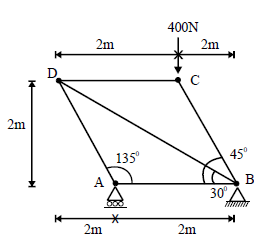Solution: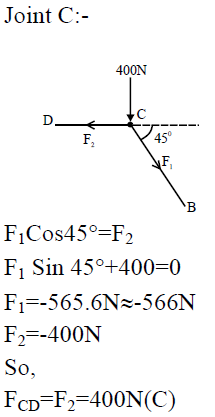QUESTION: 3

### In questions 2, force in the member BD is:-

Solution: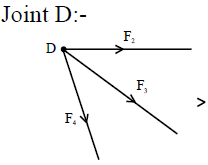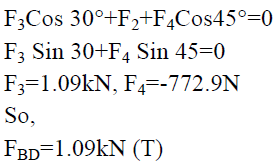QUESTION: 4

Identify the zero force members in the truss shown below :-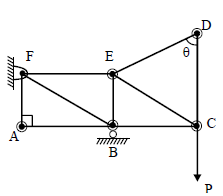Solution:
QUESTION: 5

Identify the zero force members in the truss shown below:-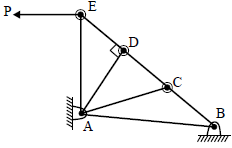Solution:
QUESTION: 6

Boy of mass 40kg is climbing a vertical pole at a constant speed. If the coefficient of friction between his palms and the pole is 0.8 (g=10m/s2), the horizontal force that he is applying on the pole is:-

Solution: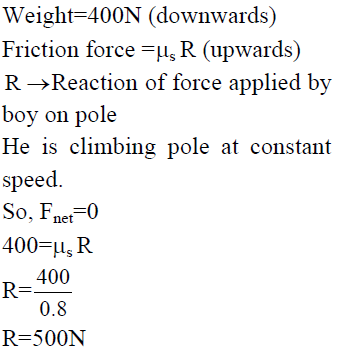QUESTION: 7

A uniform metal chain is placed on a rough table such that one end of chain hangs down over the edge of the table. When one third of its length of hangs over the edge, the chain starts sliding. Then, the coefficient of static friction is:-

Solution: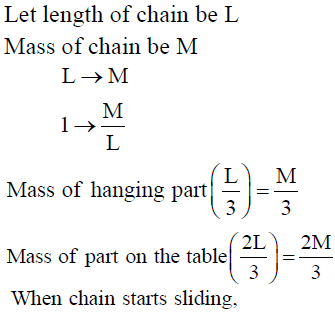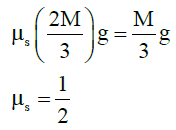QUESTION: 8

A differential pulley block has larger and smaller diameters of 100 mm and 80 mm respectively. Its velocity ratio is

Solution:
QUESTION: 9

A box is lying on an inclined plane. What is the coefficient of static friction if the box starts sliding when an angle of inclination is 60o?

Solution: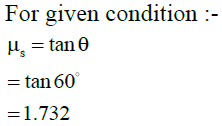QUESTION: 10

A block of mass m is placed on a surface with a vertical cross section given by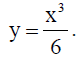If
coefficient of friction is 0.5, the maximum height above the ground at which the block can be placed without slipping is :-

Solution: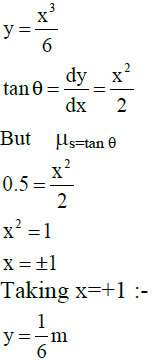QUESTION: 11

Common data for 11 & 12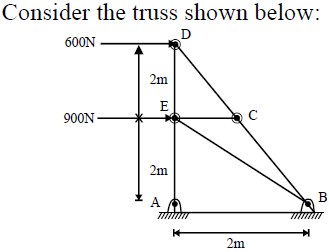Force in member EA is:-

Solution:

Joint E :-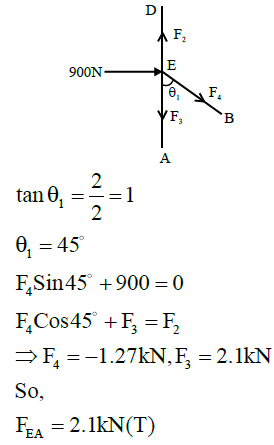QUESTION: 12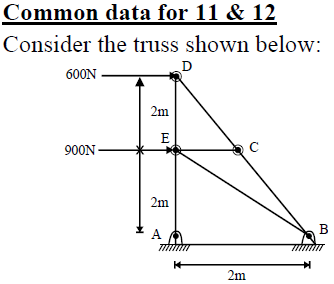Force in member DE is:-

Solution: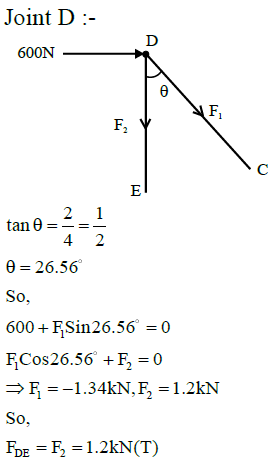QUESTION: 13

Consider the truss shown below :-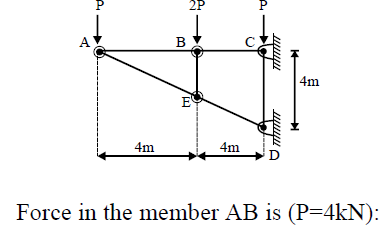Solution:

Joint A:-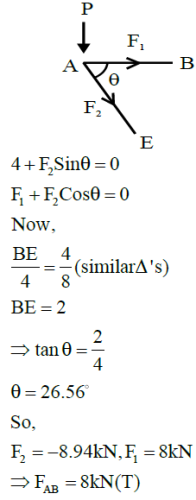QUESTION: 14

force in member BE is:-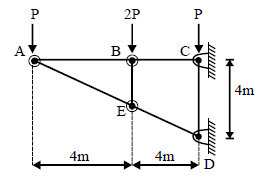Solution: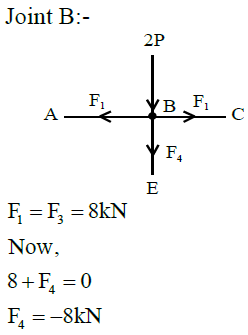QUESTION: 15

Determine the force in the member DE shown below (P= P= 4kN) :-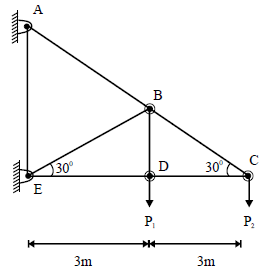Solution:

Joint C:-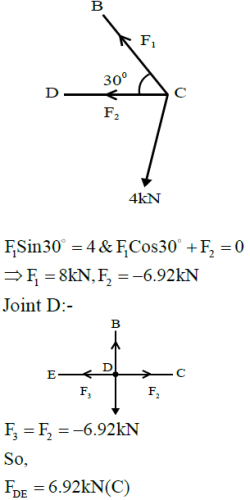QUESTION: 16

Which are the zero force members in the truss shown below:-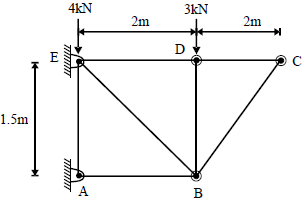Solution: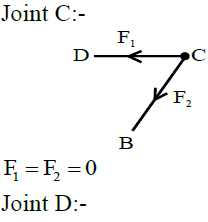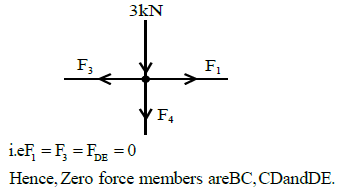QUESTION: 17

In the figure given, the system is in equilibrium. What is the maximum value that W can have if the friction
force on the 40N block cannot exceed 12 N:-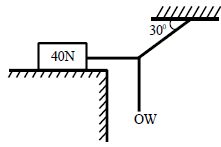Solution:
QUESTION: 18

A body of weight 64N is pushed with just enough force to start it moving across a horizontal floor and the same force continues to act afterwards. If the coefficients of static and dynamic friction are 0.6 and 0.4 respectively, the acceleration of the body will be  (g=10m/s2):-

Solution: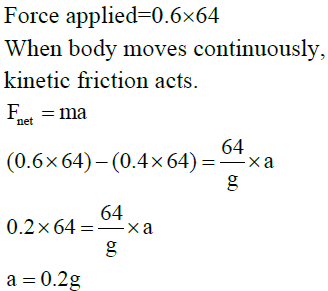QUESTION: 19

The coefficient of friction between body and surface of an inclined plane at 45º is 0.5.If g = 9.8m/s2, the acceleration of body downwards in m/s2 is:-?

Solution: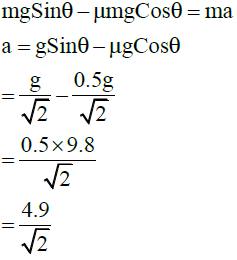QUESTION: 20

In a framed structure, as shown in the below figure, the force in the member BC is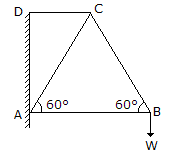Solution: